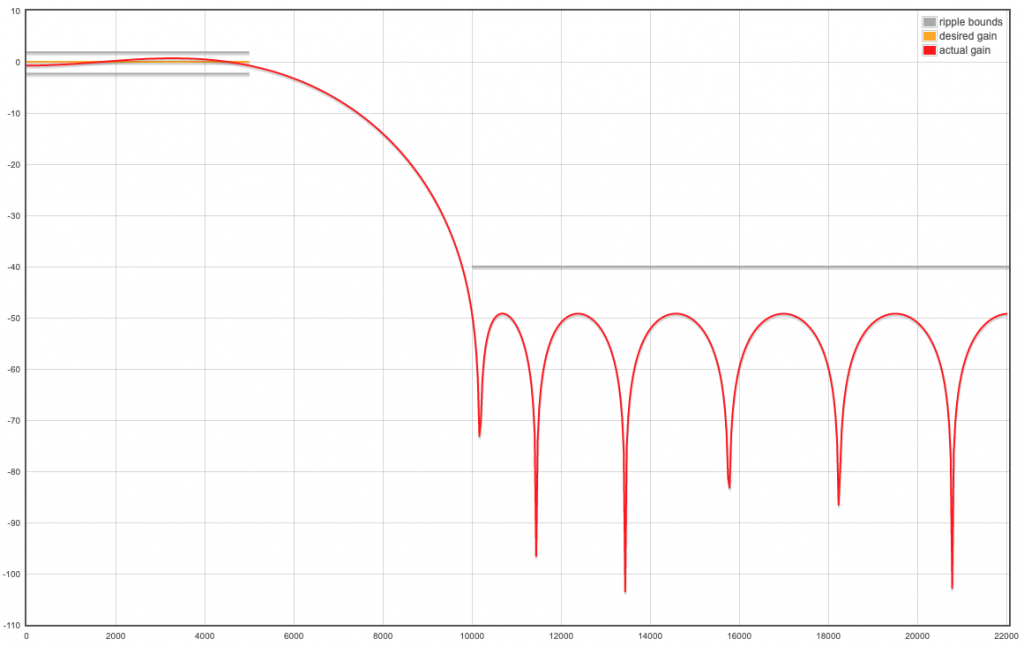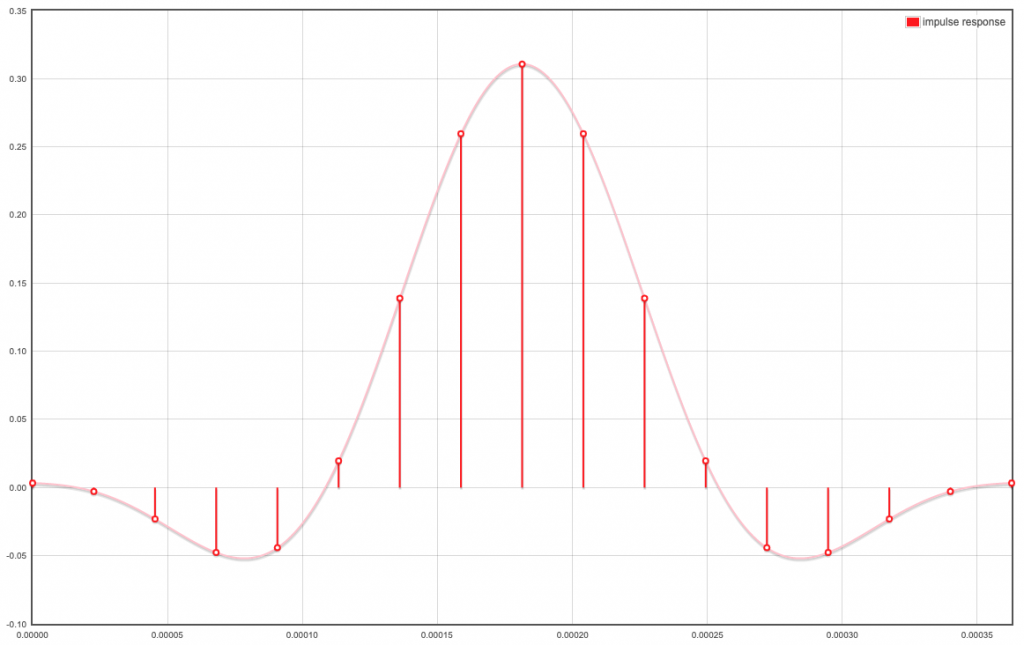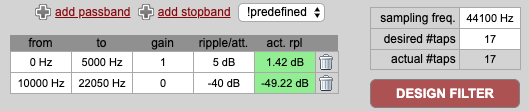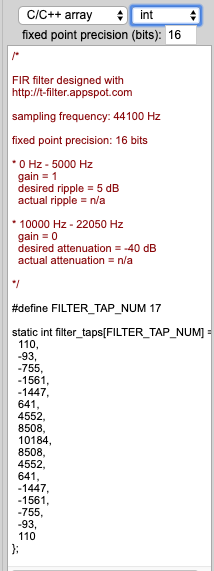APU Configuration

• @spblinux I doubt you'll get anything useful with frequency content greater than 4kHz due to spatial aliasing effects. I suggest you investigate and add a bit about that to your blog and summarize here.

4cm spacing with the 6+1 mic array is limiting factor. Generate coefficients for a 4 or 5kHz LP filter and assign to both the PREV and POST FIR filter stages. The existing POST FIR filter is notched at 5kHz and rolls off on either side.

Good luck!

• @MyAmigo some of my findings are here (to be continued); trying to get usable APU_VOC output from 6+1 mic array. - Direction detection with 440hz sine wave works fine, led on mic array showing direction of sound ...

• Here’s an updated video with the original 5kHz low pass filter in the device and a 2kHz tone. This is a much better demo than the last one.

https://photos.app.goo.gl/VvP6rKaahKcAmLwU6

• Ok so I'll share a little of what I've learned. The alternate filters seem to work. I tested a 10kHz signal on the default 5kHz LP filter and of course that was blocked. A filter with a higher cutoff frequency allowed higher frequency content through as expected. I even tested a 12kHz - 20kHz signal and the program ignored my voice while it was running. When I switched on an 18kHz source (tone generator on my phone) it got busy with bigger numbers. I found the numbers difficult to interpret since they were flying by the screen at high speed so I modified the apu demo files to generate a simple bar graph. I recorded a video >>> here <<<.

Setup...

• Using Sipeeds's 6+1 mic array
• Configured with 12-20kHz BP filter coefficients on PREV & POST FIR filters
• Fstop1 = 7kHz, Fpass1 = 12kHz, Fpass2 = 20kHz & Fstop2 = 25kHz
• I2S_SF define in apu.h changed to 54000 (54kHz) so that satisfied the 25kH Fstop
• Demo init.c uses I2S_SF instead of hardcoded value
• I disabled the VOC logic (set APU_VOC_ENABLE = 0)
• Adjusted parameters in function: apu_set_delay(4, 6, 0)... 4cm mic spacing with 6+1 array PCB; 6 mics in circle; ignore center mic

Observations:

• The relationship of the energy detected in one direction bar on the graph relative to the next doesn’t match what I thought it would.
• This is likely because the mic spacing is now too great for the test signal so the beamformer is not working.
• The Wavelength at 18kHz is 342/18000 = 0.019m = 1.9cm and we know that the spacing has to be less than ½ this value to avoid spatial aliasing (look it up) so ~1cm.
• The current spacing is 4cm so we should expect it to not work.

• The default demo came with a 5kHz LP filter.
• The wavelength of a 5kHz signal is 342/5000 = .068m = 6.8cm so the spacing should be less than 3.4cm.
• The spacing for the 6+1 array is actually 4cm so testing the default demo with a marginally 5kHz signal should produce inconsistent results.
• This probably explains why the demo was setup with a 5khz LP FIR filter... it might not work otherwise and that would make for a terrible demo

Getting there!

• @spblinux ... nice to see someone else pondering this topicI see that you have roughly the same magnitude for your coefficients as I do. I also note that the coeffecients from the BF demo code indicate a passband attentuation of ~6dB. I haven't seen a filter design tool that would also allow you to specify attenuation but a filter can be attenuated by applying a common scale factor to all coefficients. The DC gain of a filter is the sum of the coefficients, which totals 0.51532 for the BF demo. Dividing each coefficient by this number shifts the magnitude response up so there is 0 attenuation in the passband. Now we can see that a filter generated using filterDesigner needs to have a stopband attenuation of ~30dB...... so we can try different settings with our original attempt at replicating the BF coefficients and when we get this response we only need to scale the coefficients to determine values that best match the original BD demo filter.

I found that changing the Wstop parameter to 0.2928 produced a mag plot that was almost identical to the above...By then scaling the coefficients so the gain of this filter matches the original BF demo coefficients we get virtually the same mag plot as the BF demo.The RMS error between the original BF demo coefficients and these new ones after setting Wstop=0.2928 and scaling is 0.3% so that's close enough for me.

I think I can start experimenting with filter settings now.

• @MyAmigo Great! That is one more step to deal with the apu.
For all those without a copy of matlab. At http://t-filter.engineerjs.com/ very similar filter coefficients can be created:TFilter uses int16_t numbers, sdk example apu/init.c uses uint16_t.

• @manageryzy thank you. I thought the common coefficients were there to apply gain. I can confirm whether that is actually used by changing the values and seeing if it affects the performance of the hardware. Speaking of gain... there is a hardware function called audio_bf_set_audio_gain which I assume is adjusted to correct overall gain for the system so it is applied to all frequencies. Does that sound right?

I figured out how to generate a filter in Matlab using just the coefficients so I loaded the 17 coefficients from the BF demo into Matlab (after converting to fixed-point real numbers) and obtained the following impulse reponse (to confirm import)...and the resulting magnitude response...... assuming FS=48kHz. This is helpful as it suggests a Fpass of about 5kHz and an Fstop of about 10kHz if we use MatLab's filterDesigner tool. So I did that. The impulse and magnitude response for an equiripple, low pass, FIR filter with order N=16, Fpass=5kHz and Fstop=10kHz is as follows...This is very close to the filter that was generated using just the demo coefficients so I'm definitely on the right track. I note that the coefficients are about 2x those of the demo and there is an additional ripple "hump" in there but this doesn't look too bad.

I feel like I'm close to a solution but I should be able to reproduce the coefficients from the demo exactly so I would like some feedback on this.

Knowing the specific parameters that were used to determine the demo FIR coefficients would solve my problem.

Thanks... Scott

• @MyAmigo 0x03c3 is just a test value , i don't know why whese code got into drivers ... use your own coef

just generate a fir by matlab filter design tool, use fiter design tool quantilize these coeffs and copy them here

• I figured out what the fixed precision values (from -1 .. +1) are as follows for both the PREV & POST FIR vectors...I might be able to find a Matlab filter to match these coefficients but I'll be guessing so if you can provide a little more info I'll be able to move this further along.

What about the 3rd FIR filter vector?

uint16_t fir_common[] = {
0x03c3, 0x03c3, 0x03c3, 0x03c3, 0x03c3, 0x03c3,
0x03c3, 0x03c3, 0x03c3, 0x03c3, 0x03c3, 0x03c3,
0x03c3, 0x03c3, 0x03c3, 0x03c3, 0x03c3};

How is this used? You didn't show it in your earlier data flow diagram.

Thank you for your help. I really appreciate it.

Scott

• Great thanks! Can you provide the Matlab code to do this? That way I don't have to guess which filter command to use.

• signed fixed point number between -1 ~ 1

u can use matlab generate these coeffs

• I tried to answer my own questions about the number format for the cofficients. A signed 16-bit representation of a real number is available with the half-precision floating-point format. Converting the coefficients with this format results in the ones starting with 0xF being very large numbers so this can't be right.

Are there other possible formats for floating point numbers that might be used here? It would be nice to hear from a developer of the APU hardware.

• @manageryzy thank you.

This is helpful but I need to know more about the design of the FIR filters. There are 17 coefficients and I recognize that they are symmetric so that should narrow the options.

There are a variety of methods that may have been used to design a FIR filter:
https://en.wikipedia.org/wiki/Finite_impulse_response

• Window design method
• Frequency Sampling method
• Weighted least squares design
• Parks-McClellan method (also known as the Equiripple, Optimal, or Minimax method)
• Equiripple FIR filters designed using FFT algorithms

Someone must know the type of filters that are built into the hardware so please advise. If I have that information then I can use Matlab to calculate the coefficients for the filter used in the demo and then I will be able to create a filter with different properties.

Having an explaination of the values in the fir_prev_t definition ...

uint16_t fir_prev_t[] = {
0x020b, 0x0401, 0xff60, 0xfae2, 0xf860, 0x0022,
0x10e6, 0x22f1, 0x2a98, 0x22f1, 0x10e6, 0x0022,
0xf860, 0xfae2, 0xff60, 0x0401, 0x020b,
};

... would be a big help as well. Are these real-number representations?

I can't move forward without assistance.

• This post is deleted!

• This post is deleted!

• My new posts aren't showing up at end of list. Why is this happening?

• although i can't tell you the details of apu , i can tell you some pipeline about it

+----------+ 　+----------+ 　+------------+ 　+-------+ 　+-----+　+--------------------- + 　+-----+ 　+-----+
| 8ch in | - > | buffer | - > | DAS BF | -> | gain | -> | FIR | -> | DownSample | -> | FIR | -> | FFT |
+----------+　 +----------+ 　+------------+ 　+-------+ 　+------+　+---------------------+ 　+------+　+------+